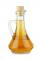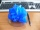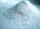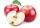Distilled water

Sea water contains 5% salt. How many distilled water should be pour to 40 kg of sea water so that the salt content is 2%. How many kilograms of 2% of sea water we get?

Result

x =  60
y =  100

Solution:

40*0.05=y*0.02
y = x+40

0.02y = 2
x-y = -40

x = 60
y = 100

Calculated by our linear equations calculator.

Leave us a comment of this math problem and its solution (i.e. if it is still somewhat unclear...):Be the first to comment!To solve this verbal math problem are needed these knowledge from mathematics:

Our percentage calculator will help you quickly calculate various typical tasks with percentages.

Next similar math problems:

1. ConcentrationHow 0.5 liters of 8% vinegar diluted to a concentration of 20 hundredths % of vinegar? How many liters of water must be pour?
2. Acid evaporationHow many kilograms of water do we have to evaporate from 100 kg of 32% acid to make it 80% concentration?
3. Copper sulphateHow much g of water do we have to add to 240 g of an 84% CuSO4 solution to produce a 60% solution? (Express the mass of crystalline CuSO4 in the original solution and in the resulting solution and compare them. )
4. CacaoCacao contains 34% filling. How many grams of filling are in 130 g cacao.
5. Bath saltsBath salt A weighs 900 grams and costs 36,40 CZK. Bath Salts B weighs 1000 grams, but costs 85 CZK. How much is a bath salt A cheaper than B one?
6. PersonsPersons surveyed:100 with result: Volleyball=15% Baseball=9% Sepak Takraw=8% Pingpong=8% Basketball=60% Find the average how many like Basketball and Volleyball. Please show your solution.
7. The ballThe ball was discounted by 10 percent and then again by 30 percent. How many percent of the original price is now?
8. SummerjobThe temporary workers planted new trees. Of the total number of 500 seedlings, they managed to plant 426. How many percents did they meet the daily planting limit?
9. Frameworks is badCalculate how many percent will increase the length of an HTML document, if any ASCII character unnecessarily encoded as hexadecimal HTML entity composed of six characters (ampersand, grid #, x, two hex digits and the semicolon). Ie. space as: &#x20;
10. PercentsHow many percents is 900 greater than the number 750?
11. Highway repairThe highway repair was planned for 15 days. However, it was reduced by 30%. How many days did the repair of the highway last?
12. Acid solutionBy adding 250 grams of a 96% solution of sulfuric acid to its 3% solution its initial concentration was changed to 25%. How many grams of 3% of the acid were used for dilution?
13. MushroomsMushrooms lose 90% by weight drying. How many fresh mushrooms are needed for 5 kg of dried mushrooms?
14. Drying applesBy drying, apples lose 85% of their weight. How many kgs of dried apples do we get from 820 kilograms of fresh?
15. Apples 14Maya bought 18 apples. She used 50% of the apples to make apple pies and 1/3 of the apples was mixed with other fruits to make salad. How many apples were left?
16. Percentages52 is what percent of 93?
17. Profit gainIf 5% more is gained by selling an article for Rs. 350 than by selling it for Rs. 340, the cost of the article is: